### Kendall’s Tau-b Correlation Coefficient

Subsections:

Kendall’s tau-b is a nonparametric measure of association based on the number of concordances and discordances in paired observations. Concordance occurs when paired observations vary together, and discordance occurs when paired observations vary differently. The formula for Kendall’s tau-b is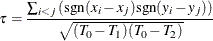where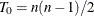,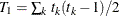, and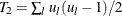. The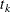is the number of tiedvalues in theth group of tiedvalues,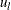is the number of tied
values in theth group of tiedvalues,is the number of observations, and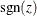is defined as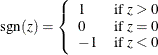PROC CORR computes Kendall’s tau-b by ranking the data and using a method similar to Knight (1966). The data are double sorted by ranking observations according to values of the first variable and reranking the observations according to values of the second variable. PROC CORR computes Kendall’s tau-b from the number of interchanges of the first variable and corrects for tied pairs (pairs of observations with equal values of X or equal values of Y).

#### Probability Values

Probability values for Kendall’s tau-b are computed by treating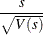as coming from a standard normal distribution where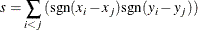and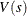, the variance of, is computed as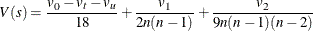where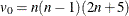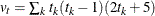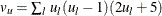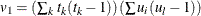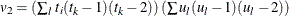The sums are over tied groups of values where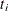is the number of tiedvalues and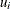is the number of tiedvalues (Noether, 1967). The sampling distribution of Kendall’s partial tau-b is unknown; therefore, the probability values are not available.